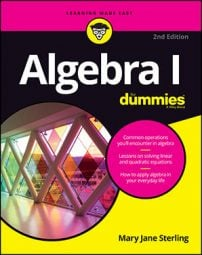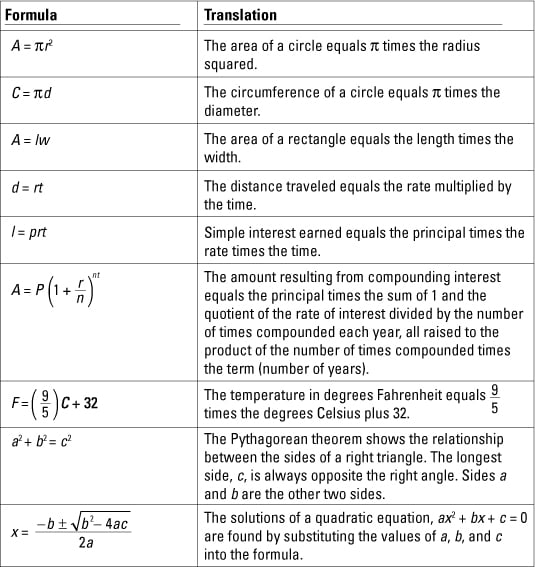##### Algebra I For DummiesAlgebra problems are easier to solve when you know the rules and formulas. Memorizing key algebra formulas will speed up your work, too. And if you know the rules of divisibility and the order of operations, you'll be able to solve algebra problems without getting stressed.

## Algebra formulas worth memorizing

Some formulas occur frequently when you’re doing algebraic manipulations and working through mathematical applications. You’ll find ways to use these algebra formulas even when you’re doing something other than algebra, such as planning a garden or a road trip. Of course, you need to know what the letters and symbols in the formula mean, so both the formulas and the explanations are in this table.## Algebra's rules of divisibility

In algebra, knowing the rules of divisibility can help you solve faster. When factoring algebraic expressions to solve equations, you need to be able to pull out the greatest factor. You also need common factors when reducing algebraic fractions. The rules of divisibility help you find the common factors and change the algebraic expressions so that they’re put in a more workable form.

• Divisibility by 2: A number is divisible by 2 if the last digit in the number is 0, 2, 4, 6, or 8.

• Divisibility by 3: A number is divisible by 3 if the sum of the digits in the number is divisible by 3.

• Divisibility by 4: A number is divisible by 4 if the last two digits in the number form a number divisible by 4.

• Divisibility by 5: A number is divisible by 5 if the last digit is 0 or 5.

• Divisibility by 6: A number is divisible by 6 if it is divisible by both 2 and 3.

• Divisibility by 8: A number is divisible by 8 if the last three digits form a number divisible by 8.

• Divisibility by 9: A number is divisible by 9 if the sum of the digits of the number is divisible by 9.

• Divisibility by 10: A number is divisible by 10 if it ends in 0.

• Divisibility by 11: A number is divisible by 11 if the sums of the alternate digits are different by 0, 11, 22, or 33, or any two-digit multiple of 11. In other words, say you have a six-digit number: Add up the first, third, and fifth digits — the odd ones. Then add the digits in the even places — second, fourth, and sixth. Then subtract the smaller of those totals from the larger total, and if the answer is a multiple of 11, the original number is divisible by 11.

• Divisibility by 12: A number is divisible by 12 if the last two digits form a number divisible by 4 and if the sum of the digits is divisible by 3.

## Order of operations in algebra

Solve algebra problems correctly by following the order of operations. When performing more than one operation on an algebraic expression, work out the operations and signs in the following order:

1. First calculate powers and roots.

2. Then perform all multiplication and division.

3. Finish with addition and subtraction.

If you have more than two operations of the same level, do them in order from left to right, following the order of operations.

For example, to solve 24 ÷ 3 + 11 – 32 × 2, you would

1. First calculate powers and roots.

This problem doesn’t have any roots, but it does have one power, 32. You know that 32 = 3 × 3 = 9. Substitute 9 into the problem, and you get 24 ÷ 3 + 11 – 9 × 2.

2. Then perform all multiplication and division.

Working left to right, 24 ÷ 3 = 8 and 9 × 2 = 18. Substitute those numbers into the problem, and you have 8 + 11 – 18.

3. Finish with addition and subtraction.

So, you end up with 8 + 11 – 18 = 19 – 18 = 1.